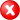#Boost C++ Libraries

...one of the most highly regarded and expertly designed C++ library projects in the world.

##### centroid

Calculates the centroid of a geometry.

###### Description

The free function centroid calculates the geometric center (or: center of mass) of a geometry. It uses the default strategy, based on the coordinate system of the geometry.

###### Synopsis

```template<typename Geometry, typename Point>
void centroid(Geometry const & geometry, Point & c)```

###### Parameters

Type

Concept

Name

Description

Geometry const &

Any type fulfilling a Geometry Concept

geometry

A model of the specified concept

Point &

Any type fulfilling a Point Concept

c

The calculated centroid will be assigned to this point reference

Either

`#include <boost/geometry.hpp>`

Or

`#include <boost/geometry/algorithms/centroid.hpp>`

###### Conformance

The function centroid implements function Centroid from the OGC Simple Feature Specification.

###### Behavior

Case

Behavior

Point

Returns the point itself as the centroid

Multi Point

Calculates centroid (based on average)

linear (e.g. linestring)

Calculates centroid (based on weighted length)

areal (e.g. polygon)

Calculates centroid

Empty (e.g. polygon without points)

Throws a centroid_exception

Cartesian

Implemented

Spherical

Calculates the centroid as if based on Cartesian coordinates

###### Supported geometries

2D

3D

PointSegmentBoxLinestringRingPolygonMultiPointMultiLinestringMultiPolygonLinear

###### Example

Shows calculation of a centroid of a polygon

```#include <iostream>
#include <list>

#include <boost/geometry.hpp>
#include <boost/geometry/geometries/point_xy.hpp>
#include <boost/geometry/geometries/polygon.hpp>

int main()
{
typedef boost::geometry::model::d2::point_xy<double> point_type;
typedef boost::geometry::model::polygon<point_type> polygon_type;

polygon_type poly;
"POLYGON((2 1.3,2.4 1.7,2.8 1.8,3.4 1.2,3.7 1.6,3.4 2,4.1 3,5.3 2.6,5.4 1.2,4.9 0.8,2.9 0.7,2 1.3)"
"(4.0 2.0, 4.2 1.4, 4.8 1.9, 4.4 2.2, 4.0 2.0))", poly);

point_type p;
boost::geometry::centroid(poly, p);

std::cout << "centroid: " << boost::geometry::dsv(p) << std::endl;

return 0;
}
```

Output:

```centroid: (4.04663, 1.6349)```

Note that the centroid might be located in a hole or outside a polygon, easily.